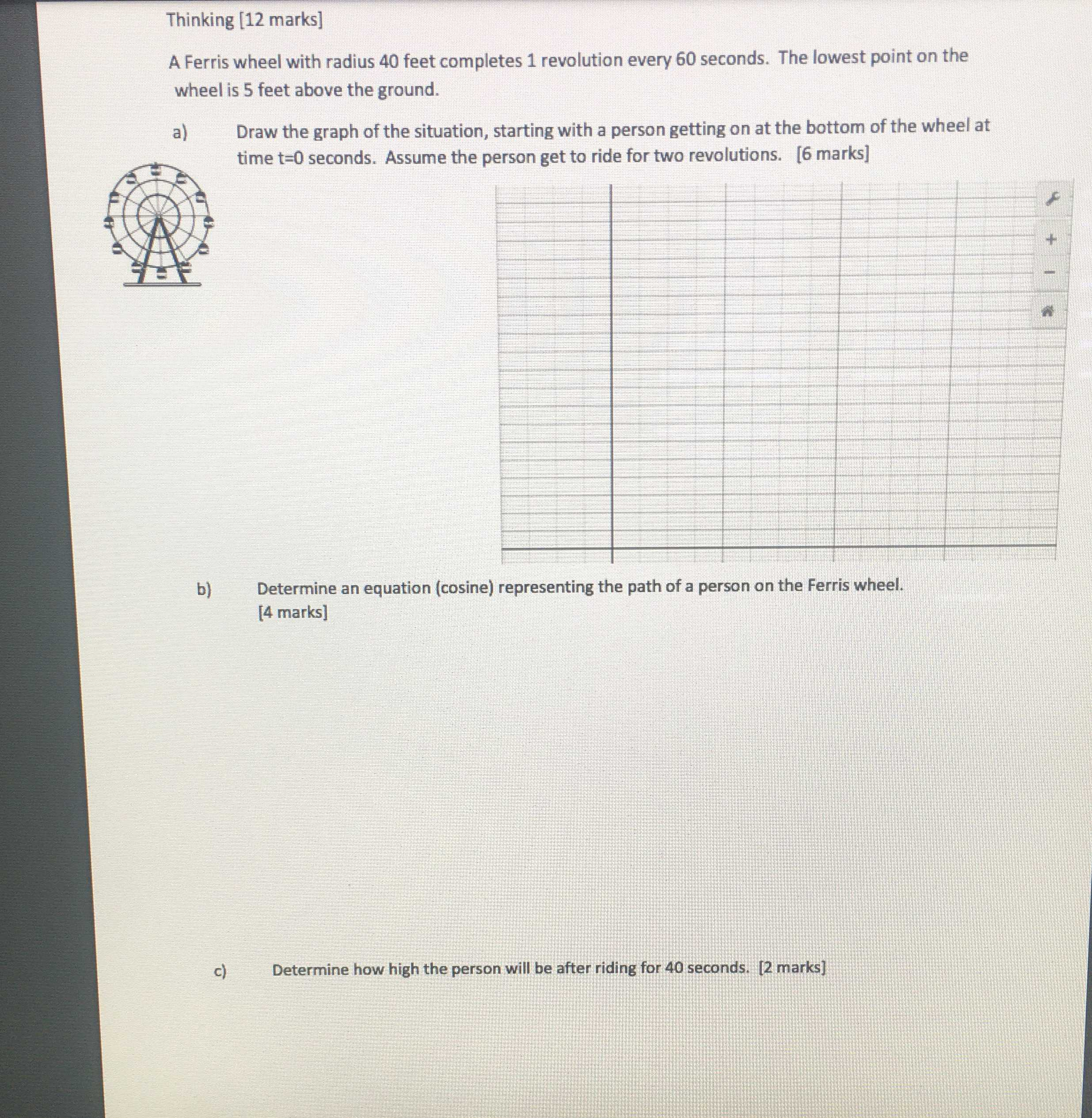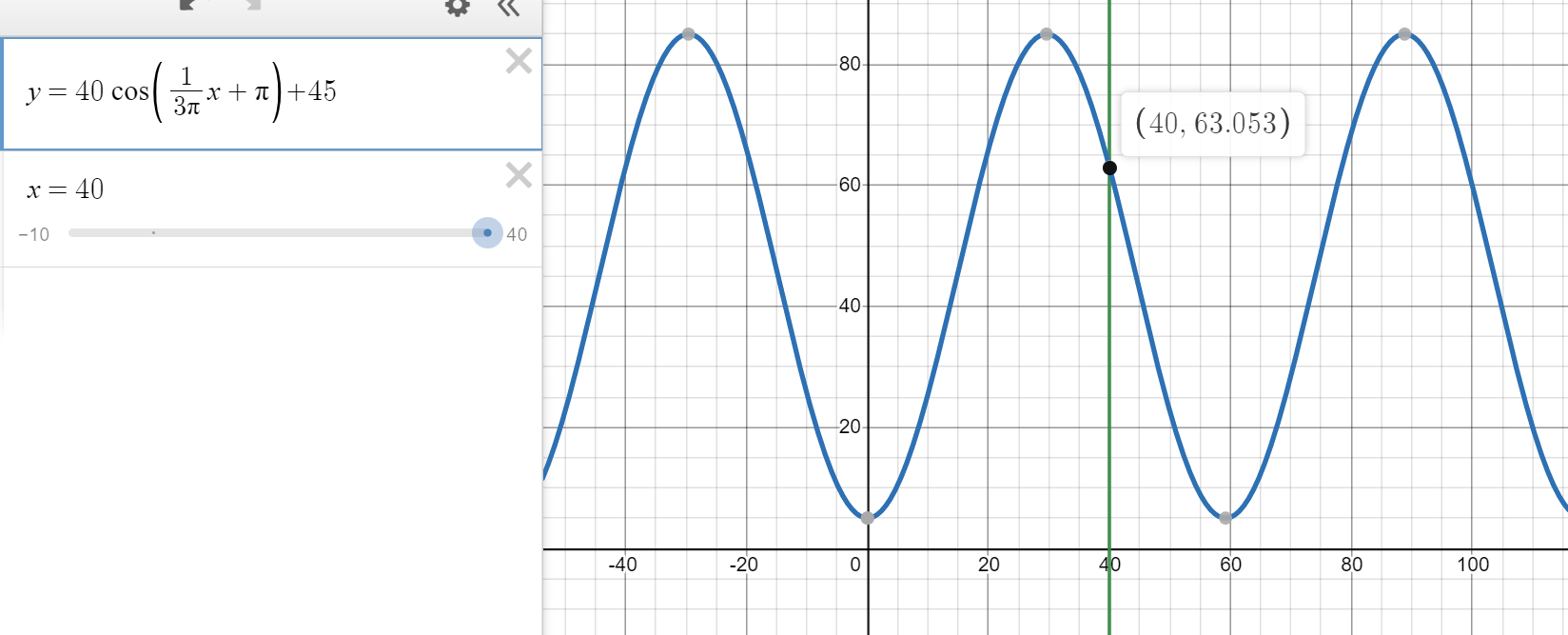### ¿Todavía tienes preguntas de matemáticas?

Pregunte a nuestros tutores expertos
Algebra
PreguntaA Ferris wheel with radius $$40$$ feet completes $$1$$ revolution every $$60$$ seconds. The lowest point on the wheel is $$5$$ feet above the ground.

a) Draw the graph of the situation, starting with a person getting on at the bottom of the wheel at time t=0 seconds. Assume the person get to ride for two revolutions. [6 marks]

b) Determine an equation (cosine) representing the path of a person on the Ferris wheel. [4 marks]

c) Determine how high the person will be after riding for $$40$$ seconds. [2 marks]$$y = 40 \cos ( \frac { 1 } { 3 \pi } x + \pi ) + 45$$
$$x = 40$$